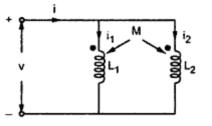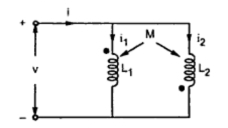### Inductive Coupling in Parallel

When two inductors having self inductance L1 and L2 are coupled in parallel, we have two kinds of connections as follows.
1.1 Parallel Aiding
Consider parallel coupling of two inductors as shown in Fig.1.Fig. 1

Applying KVL to both loops, we get,
- jω L1 i1 - jω M i2 + v = 0
- jω L2 i2 - jωM i1 + v = 0
i.e.                 v = jωL1 i1 + jωM i2               ...............(1)
v = jωL2 i2 + jωM i1                 ..............(2)
We have,        jωL1 . i1 + jωM .  i2 = jωL2 .  i2 + jω M . i1
But                  i = i1 i2
i.e.                    i2 = i -  i1
Putting value of i2 in above equation, we get
...                      jωL1 i1 + jω M(i - i1) = jω L2 ( i - i1) + jω M i1
...                       i1 (L1 + L2 - 2M) = jω i(L2 -M)
...                       i1= {(L2 - M)/(L1 + L2 -2M)} i
Similarly,            i2 = {(L1 -M)/(L1 + L2 - 2M)} i
Putting values of i1and i2 in equation (1), we get,
If L is effective inductance of parallel combination then,
v = jωLeff . i                              ............(4)
Comparing equations (3) and (4) we have
Leff  = (L1 L2 - M2)/(L1 + L2 - 2M)

1.2 Parallel Opposing
Consider two inductors connected in parallel as shown in Fig.2.Fig. 2

Applying KVL to both loops, we get,
- jω L1 i1 + jω M i2 + v = 0
- jω L2 i2 + jωM i1 + v = 0
i.e.             jωL1 i1 - jωM i2 = v                      ...............(5)
jωL2 i2 - jωM i1 = v                      ..............(6)
We have,    jωL1 i1 - jωM  i2 = jωL2  i2 - jω M  i1
But             i = i1 i2
...                i2 = i -  i1
Substituting value of i2 in above equation, we get
jωL1 i1 + jω M(i - i1) = jω L2 ( i - i1) - jω M i1
...                jω i1 (L1 + L2 + 2M) = jω i(L2 + M)
...                i1= {(L2 + M)/(L1 + L2 + 2M)} i
Similarly,     i2 = {(L1 + M)/(L1 + L2 + 2M)} i
Putting values of and in equation (1) we get,
If L is effective inductance of parallel combination then,
v = jωLeff . i                                 ............(8)
Comparing equations (7) and (8) we have
Leff  = (L1 L2 - M2)/(L1 + L2 + 2M)